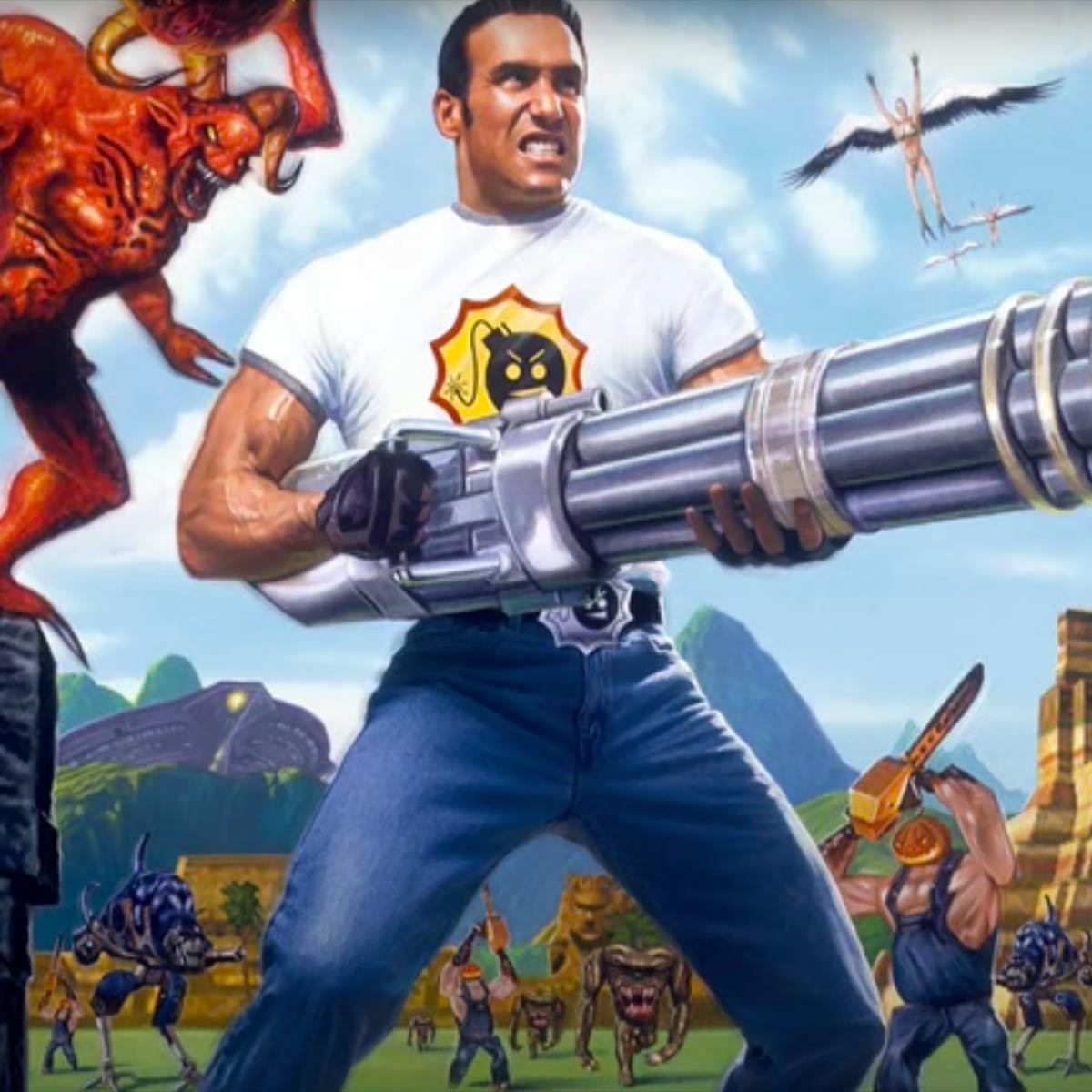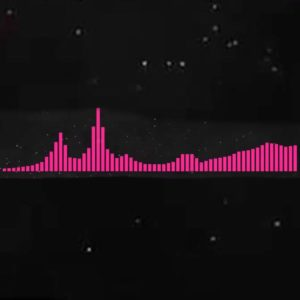# The Grand Cathedral (Serious Sam)

10 September 2020
4
• ##### TYPE
0
(0)

f dsapoi u ioiy
[3f] dsapoi [3u] iopi [3p] [3a] pdapasi opasapoio
[3f] dsapoi [3u] ioiy
[3f] dsapoi [u3] iopi [3p] [3a] pdapasi opasapoio
[3h] [3h] [3g] [3g] j [3k] [3k] [3j] [3j] kj [3h] [3h] [3g] [3g]
d [3g] [3g] [3h] [3h] j jhg jhg jhg g g g

Rate This Music Sheet:

Average rating 0 / 5. Vote count: 0

No votes so far! Be the first to rate this music sheet.

Thank you for rating this song!

If you have any specific feedback about how to improve this music sheet, please submit this in the box below.

• A song by

The Grand Cathedral (Serious Sam) is a song by Damjan Mravunac. Use your computer keyboard to play The Grand Cathedral (Serious Sam) music sheet on Virtual Piano. This is an Easy song and requires practice. The song The Grand Cathedral (Serious Sam) is classified in the genre of Songs From Games on Virtual Piano. You can also find other similar songs using Instrumental, Inspirational.

## Related

•u r y e r y | e r t y e u r y e r y | e r t y y 9 9 0 0 0 e w Q Q Q Q w r t r 9 0 t r w w r e w Q 0 | f a d p a d p a s d p f a d p a d p a s d d y y u u u p o I I I o a s a y u s a o o a p a d f | u u u u I I I I I I I a I p o p p p p d p s a p o o | [h f s o u t w 0 8 5 3 1] u u u u I I I I I I I a I p o p p p p d p s a p o f | 0 Q w o o o p I y s a p [o0] Q w o o o p I y s a p [o0] Q w o o o p I y s a p o o o o o o a p o I d f | i t Y E t Y E t T Y E i t Y E t Y E t T Y Y | Y Y i i i P O o o o O s S s Y i S s O O s P s D g q w W O O O P o Y S s P [Oq] w W O O O P o Y S s P [Oq] w W O O O P o Y S s P O O O O O O s P O o D g | q w W O O O P o Y S s P [Oq] w W O O O P o Y S s P [Oq] w W O O O P o Y S s P O O O O O O s J H h Z c
Level: 4
Easy

#### Donny Osmond

•e T T T e T T T S [I7] e S [a0] p a T [f6] f f f [fT] S S a [a9] p T S [I7] e S [a0] p a T [f6] f f f [fT] S S a [a9] p S S a p [a7] u [eS] S a p [a0] u [TS] S a p [a6] u [TS] S a p [a9] u T S [I7] e S [a0] p a T [f6] f f f [fT] S S a [a9] p T [d7] d d d [ed] d d d [f0] S T [d6] d d d [dT] d d d [f9] S T [a7] p a p [ea] p a p [a0] T p [a6] p a p [aT] p a p a d d d d [f7] S S [eS] d S [a0] [dT] d d d [f6] d S [TS] d S [a9] [dT] d d d [f7] d S [eS] d S [a0] [dT] d d d [f6] d S [TS] d S [a9] T a p [a7] p [pe] [a0] p [pT] p [a6] S S S [TS] a p p [a9] p [pT] [a7] p [pe] [a0] p [pT] [a6] S S S [TS] a p p a p p S [I7] e S [a0] p a T [f6] f f f [fT] S S a [a9] p T S [I7] e S [a0] p a T [f6] f f f [fT] S S a [a9] p S S a p [a7] u [eS] S a p [a0] u [TS] S a p [a6] u [TS] S a p [a9] u T S [I7] e S [a0] p a T [f6] f f f [fT] S S a [a9] p T S a [S7] S e S a [S0] S T S a [S6] S a [TS] a S a [S9] S T S a [a7] u e S p [a0] u T S p [a6] a [aT] S a p [a9] u T S p [f7] S a e S S [f0] S a T S S [S6] S S [TS] S S f S a p [S7] a a p [ea] p a [a0] a p [aT] I [a6] S a p [aT] p a p [a9] p a p [aT] a p [a7] p [pe] [a0] p [pT] p [a6] S S S [TS] a p p [a9] p [pT] [a7] p [pe] [a0] p [pT] [a6] S S S [TS] a p p a p p S [I7] e S [a0] p a T [f6] f f f [fT] S S a [a9] p T S [I7] e S [a0] p a T [f6] f f f [fT] S S a a p S S a p [a6] u [TS] S a p [a9] u [TS] S a p [a7] u [eS] S a p [a0] u T S [I6] T S [a9] p a T [f7] f f f [fe] S S a [a0] p T e T T T e T T T

Level: 4
Easy

#### Arizona Zervas

•i i i o O s P P O P s P O i i i o O i s P O P s P O g g g h H c c c Z l | l J l v v v x V c g g g h H c c c Z l | H J l J l J H h H g g g g h H c c c Z l | l J l v v v x V c g g g h H c c c Z l | H J l J l J H h H g s O i t w q
Level: 3
Easy